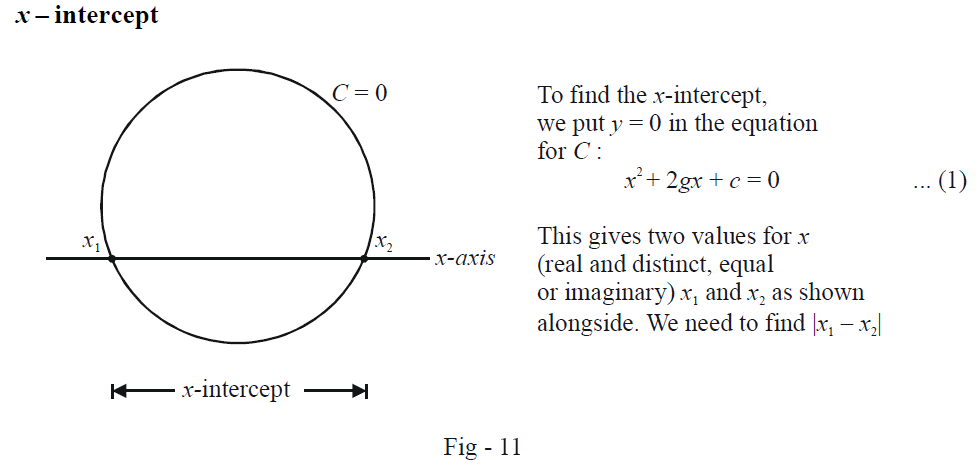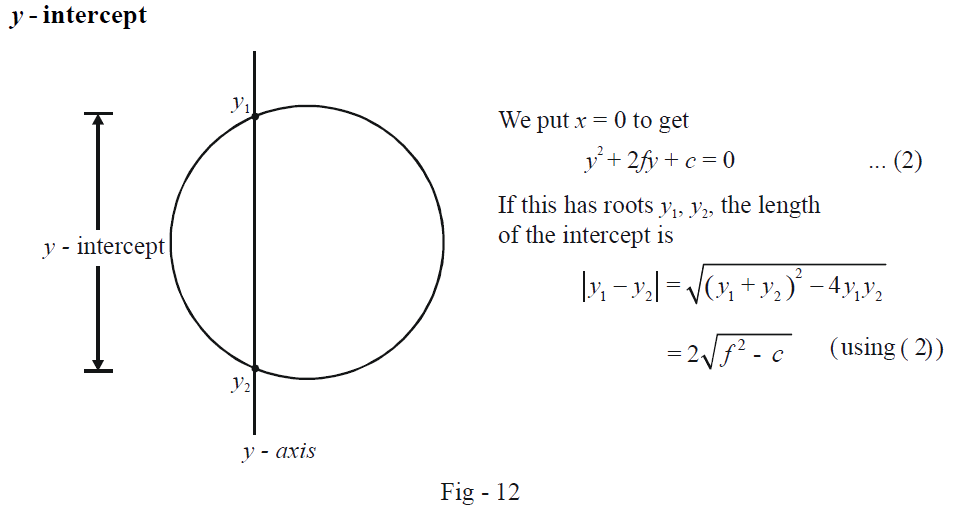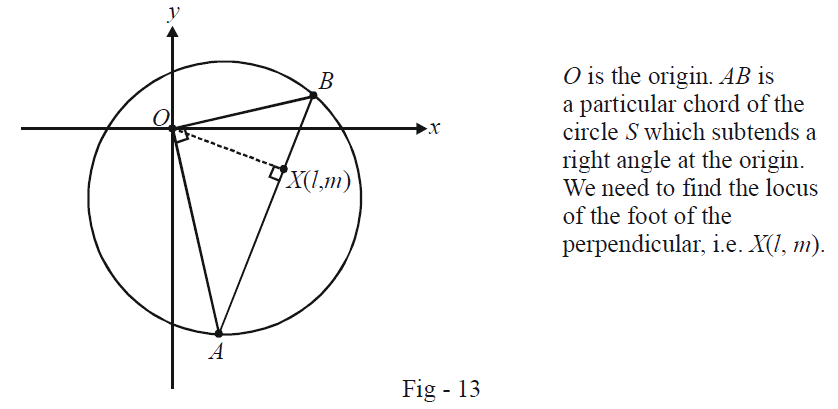# Jee Examples on Equations of Circles Set-3

Go back to  'Circles'

Example -13

Given the circle \begin{align}C:{x^2} + {y^2} + 2gx + 2fy + c = 0\end{align}, find the intercepts that it makes on the $$x$$-axis and $$y$$-axis.

Solution: We did a similar case in Example - 8 by solving simultaneously the equation of the circle and the line on which the intercept is required. Here, we’ll proceed analogously.

x – interceptFrom (1), we have \begin{align}{x_1} + {x_2} = - 2g\,\,{\rm{and}}\,\,{x_1}{x_2} = c.\end{align}

Thus,

\begin{align}\left| {{x_1} - {x_2}} \right| &= \sqrt {{{\left( {{x_1} + {x_2}} \right)}^2} - 4{x_1}{x_2}} \\&= 2\sqrt {{g^2} - c} \end{align}

y - interceptThus, the intercepts are of length \begin{align}2\sqrt {{g^2} - c} \,\,{\rm{and}}\,\,2\sqrt {{f^2} - c} \end{align} respectively. Obviously, if \begin{align}{g^2} < c\end{align} the circle and the x–axis do not touch/intersect; if  $${{f}^{2}}<c$$  the circle and the y–axis do not touch or intersect.

Example -14

What values can the variable a take so that the point $$(a – 1, a + 1)$$ lies inside the circle \begin{align}{x^2} + {y^2} - 12x + 12y - 62 = 0\end{align} but outside the circle \begin{align}{x^2} + {y^2} = 8\end{align}.

Solution: We will use the general result that we derived in Example - 6 earlier (refer). Specifically, if $$S = 0$$ is the equation of circle and $$P (x_1, y_1)$$ be any point, then

\begin{align} &S\left( {{x_1},{y_1}} \right) < 0 \qquad \Rightarrow \qquad P\,\,{\text{lies inside}}\,S\\ &S\left( {{x_1},{y_1}} \right) > 0 \qquad \Rightarrow \qquad P\,\,{\text{lies outside}}\,S \end{align}

Using these relations for the current case, we obtain and

\begin{align} {\left( {a - 1} \right)^2} + {\left( {a + 1} \right)^2} - &12\left( {a - 1} \right) + 12\left( {a + 1} \right) - 62 < 0 \qquad \qquad ...(1)\\ & \Rightarrow \qquad 2{a^2} - 36 < 0\\ & \Rightarrow \qquad - 3\sqrt 2 < a < 3\sqrt 2 \qquad \qquad \quad \quad \;\; ...(i)\\ and\;{\left( {a - 1} \right)^2} +& {\left( {a + 1} \right)^2} - 8 > 0 \qquad \qquad \qquad \qquad \quad \quad \;\; ...(2)\\ & \Rightarrow \qquad 2{a^2} - 6 > 0\\ & \Rightarrow \qquad a > \sqrt 3 \,\,{\rm{or}}\,\,a < - \sqrt 3 \qquad \qquad \quad ...(ii) \end{align}

The intersection of (i) and (ii) gives us the required values of $$a$$ as

$a \in \left( { - 3\sqrt 2 , - \sqrt 3 } \right) \cup \left( {\sqrt 3 ,\,\,3\sqrt 2 } \right)$

Example -15

Find the locus of the foot of the perpendicular drawn from the origin upon any chord of a circle \begin{align}S \equiv {x^2} + {y^2} + 2gx + 2fy + c = 0\end{align} which subtends a right angle at the origin.

Solution:    The situation is depicted graphically in the figure below to make things clearer:Observe that the equation of the chord $$AB$$ can be written as

\begin{align} & \frac{{y - m}}{{x - l}} = \frac{{ - l}}{m} & & \left( {\because AB \bot OX} \right) \hfill \\ \Rightarrow \qquad & \; lx + my = {l^2} + {m^2} \hfill \\ \end{align}

Now, if we homogenize the equation of $$S$$ using the equation of the chord $$AB$$, what we’ll get is the equation of the pair of straight lines $$OA$$ and $$OB$$ (as discussed in the last chapter on straight lines). This is what we proceed to do:

\begin{align}{x^2} + {y^2} + 2gx\left( {\frac{{{l} x + my}}{{{l^2} + {m^2}}}} \right) + 2fy\left( {\frac{{lx + my}}{{{l^2} + {m^2}}}} \right) + c{\left( {\frac{{lx + my}}{{{l^2} + {m^2}}}} \right)^2} = 0 \qquad \qquad \qquad ...(1)\end{align}

This is the joint equation of $$OA$$ and $$OB$$, since $$OA$$ and $$OB$$ need to be at right angles, we impose the appropriate constraint for perpendicularity on (1):

\begin{align} &{\rm{Coeff}}{\rm{. of }}{x^2} + {\rm{coeff}}.\,{\rm{of}}\,{y^2} = 0\\ &\left\{ {1 + \frac{{2gl}}{{{l^2} + {m^2}}} + \frac{{c{l^2}}}{{{{\left( {{l^2} + {m^2}} \right)}^2}}}} \right\}\,\, + \,\,\left\{ {1 + \frac{{2fm}}{{{l^2} + {m^2}}} + \frac{{c{m^2}}}{{{{\left( {{l^2} + {m^2}} \right)}^2}}}} \right\} = 0\\ \Rightarrow \qquad & 2 + \frac{{2gl}}{{{l^2} + {m^2}}} + \frac{{2fm}}{{{l^2} + {m^2}}} + \frac{{c\left( {{l^2} + {m^2}} \right)}}{{{{\left( {{l^2} + {m^2}} \right)}^2}}} = 0\\ \Rightarrow \qquad & {l^2} + {m^2} + gl + fm + \frac{c}{2} = 0 \end{align}

This is the equation of a circle. To be more conventional, we should use $$(x, y)$$ instead of the variables $$l$$ and $$m$$.

Thus, the required locus is

\begin{align}{x^2} + {y^2} + gx + fy + \frac{c}{2} = 0\end{align}

## TRY YOURSELF - I

Q. 1    Find the greatest and least distances of the point $$P (10, 7)$$ from the circle \begin{align}{x^2} + {y^2} - 4x - 2y - 20 = 0\end{align}

Q. 2  Through the origin $$O$$, a straight line is drawn to cut the line \begin{align}y = mx + c\end{align} at $$P$$. If $$Q$$ is a point on this line such that \begin{align}OP \cdot OQ = {\lambda ^2}\end{align}, show that the locus of $$Q$$ is a circle passing through the origin.

Q. 3    What is the area of an equilateral triangle inscribed in the circle \begin{align}{x^2} + {y^2} + 2gx + 2fy + c = 0?\end{align}

Q. 4    Determine the equation of the circle passing through the points $$(1, 2)$$ and $$(3, 4)$$ and touching the line \begin{align}3x + y - 3 = 0.\end{align}

Q. 5    A circle whose centre is the point of intersection of the lines \begin{align}2x - 3y + 4 = 0\end{align} and \begin{align}3x + 4y - 5 = 0\end{align} passes through the origin. Find its equation.

Q. 6    Find the equation of the circle which touches the $$x$$-axis and two of whose diameters lie along \begin{align}2x - y - 5 = 0\,\,{\rm{and}}\,\,3x - 2y - 8 = 0\end{align}

Q. 7    Find the equation of the circumcircle of an equilateral triangle two of whose vertices are $$(–1, 0)$$ and $$(1, 0)$$ and the third vertex lies above the $$x$$–axis.

Q. 8    Find the equation of the circle passing through $$(1,0)$$ and $$(0,1)$$ and having the smallest possible radius.

Q. 9    The equations of the sides of a quadrilateral are given by  \begin{align}{L_r} = {a_r}x + {b_r}y + {c_r} = 0\,\,\left( {r = 1,2,3,4} \right).\end{align} If the quadrilateral is concyclic, show that

$\frac{{{a}_{1}}{{a}_{3}}-{{b}_{1}}{{b}_{3}}}{{{a}_{2}}{{a}_{4}}-{{b}_{2}}{{b}_{4}}}=\frac{{{a}_{1}}{{b}_{3}}+{{a}_{3}}{{b}_{1}}}{{{a}_{2}}{{b}_{4}}+{{a}_{4}}{{b}_{2}}}$

Q. 10    Find the point on the straight line \begin{align}y = 2x + 11\end{align} which is nearest to the circle

\begin{align}16\left( {{x^2} + {y^2}} \right) + 32x - 8y - 50 = 0\end{align}